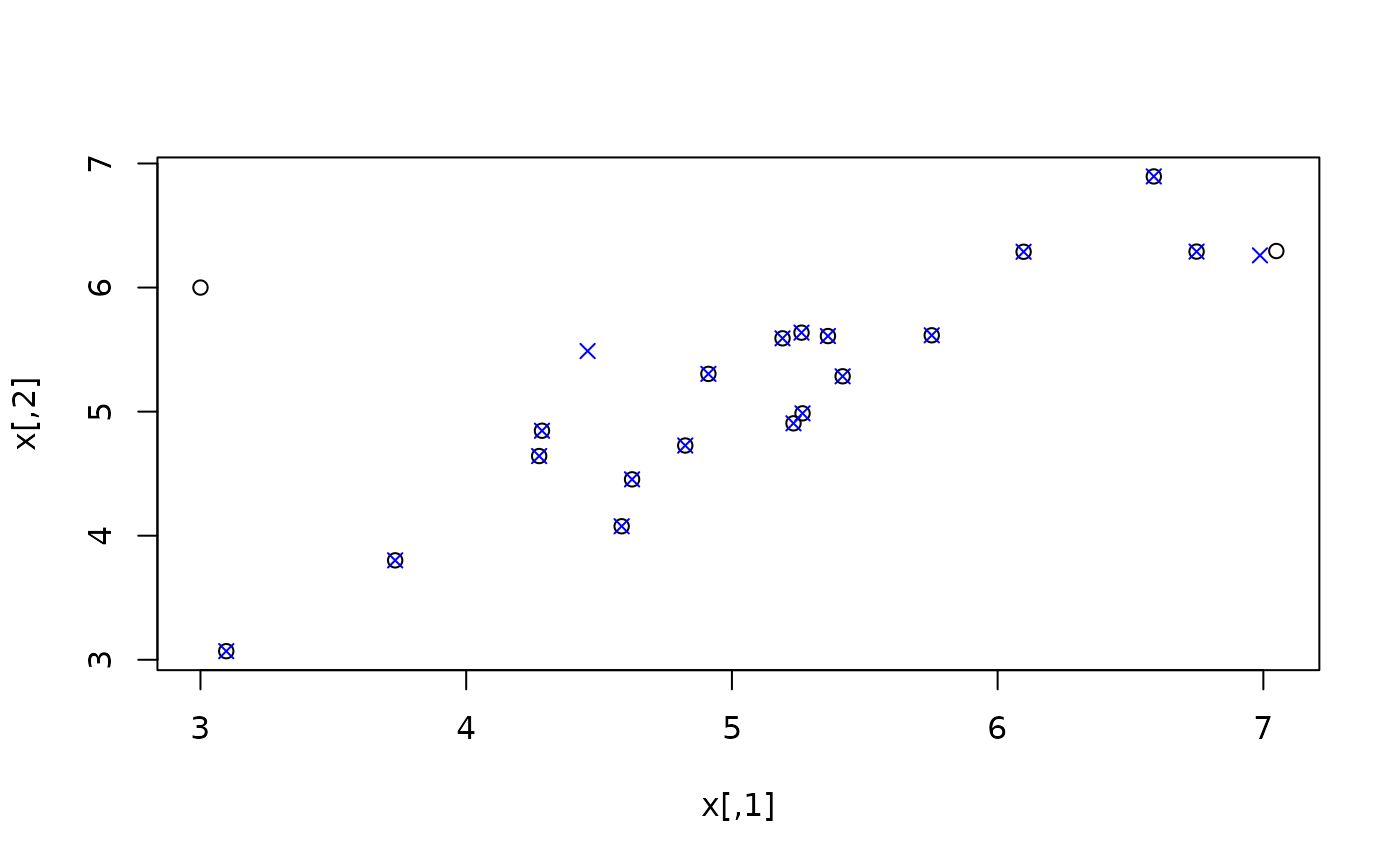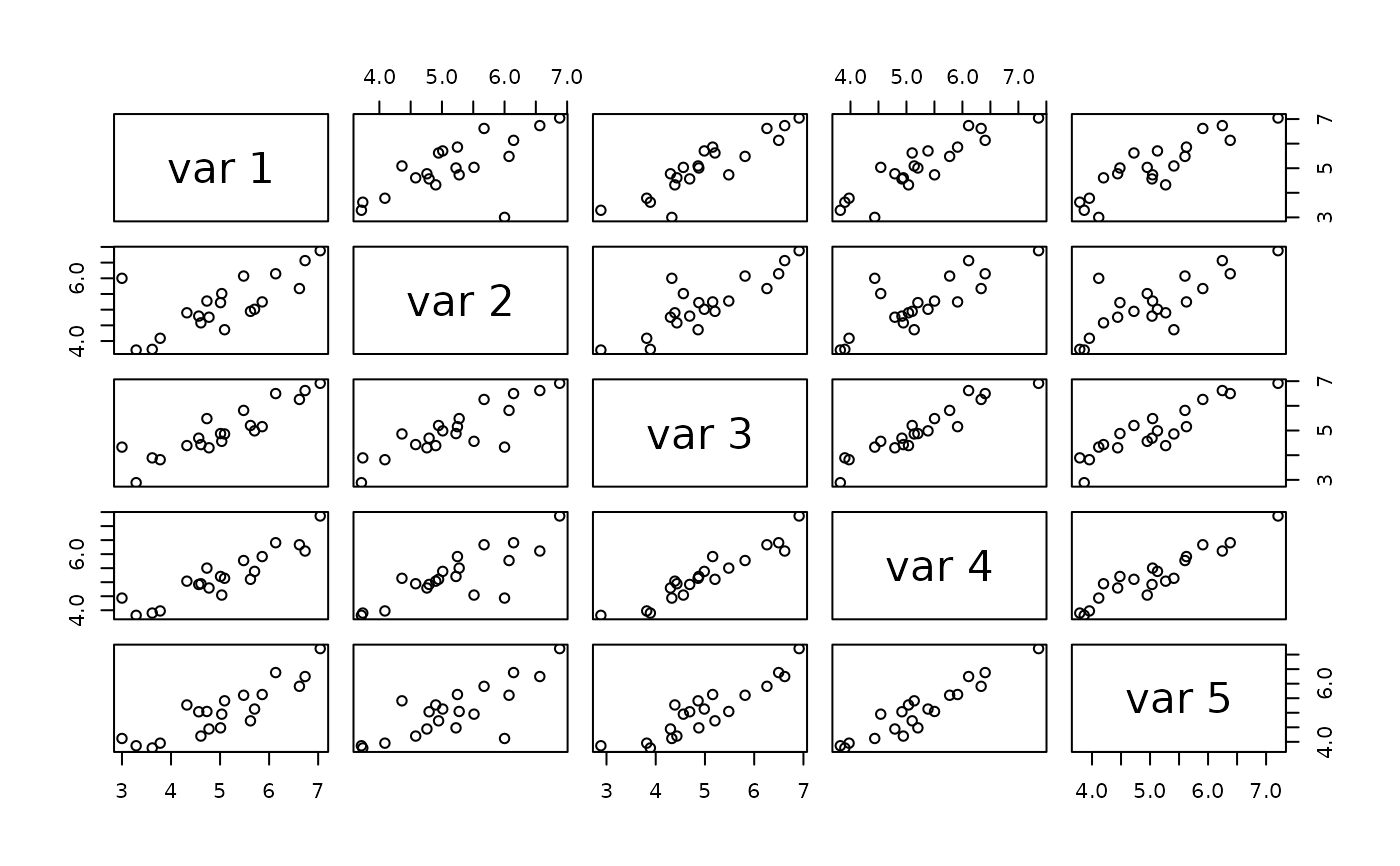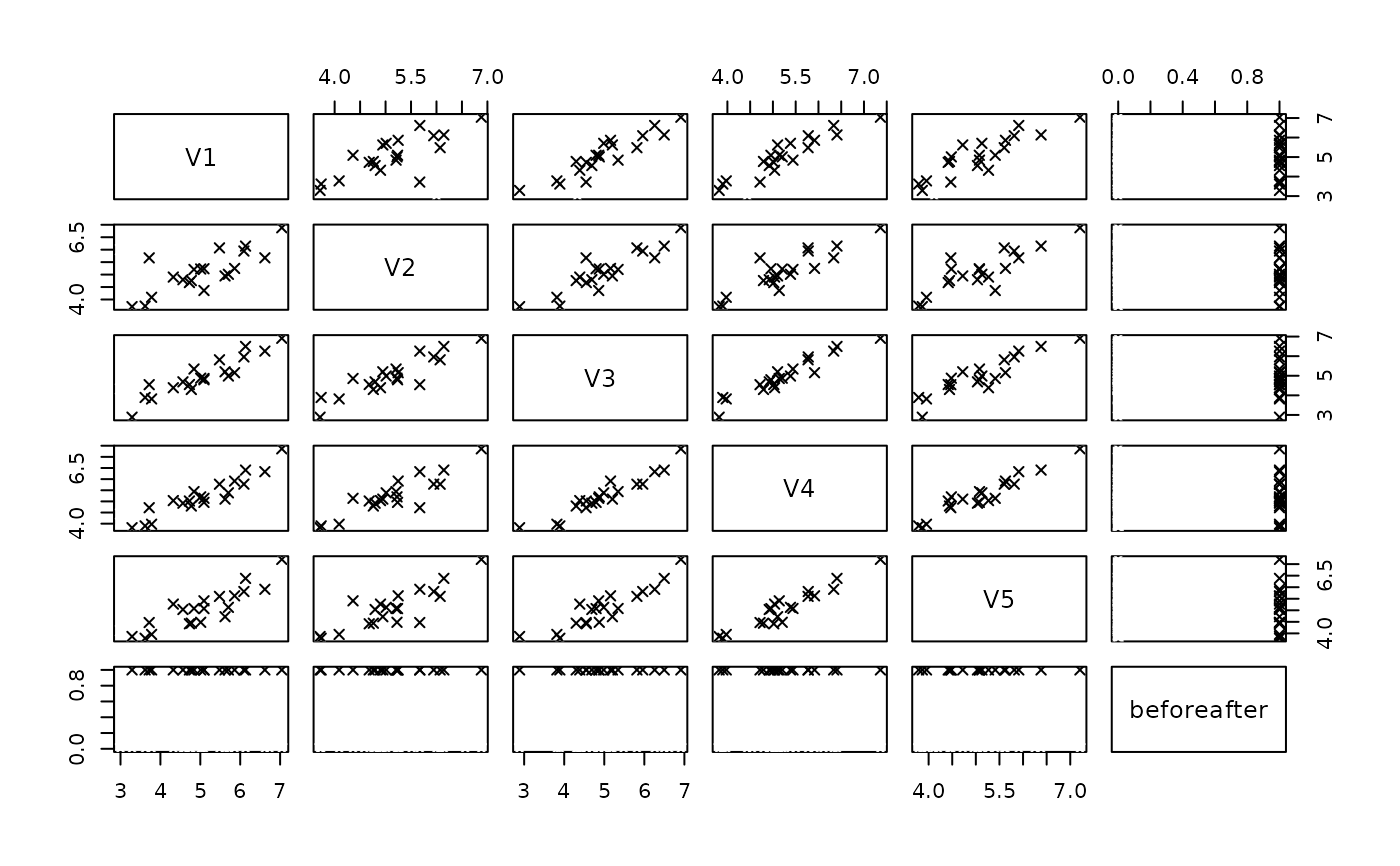Imputation and detection of outliers

mvTopCoding(x, maha = NULL, center = NULL, cov = NULL, alpha = 0.025)

## Arguments

x

an object coercible to a data.table containing numeric entries

maha

squared mahalanobis distance of each observation

center

center of data, needed for calculation of mahalanobis distance (if not provided)

cov

covariance matrix of data, needed for calcualtion of mahalanobis distance (if not provided)

alpha

significance level, determining the ellipsoide to which outliers should be placed upon

## Value

the imputed winsorized data

## Details

Winsorizes the potential outliers on the ellipsoid defined by (robust) Mahalanobis distances in direction to the center of the data

## Author

Johannes Gussenbauer, Matthias Templ

## Examples

set.seed(123)
x <- MASS::mvrnorm(20, mu = c(5,5), Sigma = matrix(c(1,0.9,0.9,1), ncol = 2))
x[1, 1] <- 3
x[1, 2] <- 6
plot(x)
ximp <- mvTopCoding(x)
points(ximp, col = "blue", pch = 4)# more dimensions
Sigma <- diag(5)
Sigma[upper.tri(Sigma)] <- 0.9
Sigma[lower.tri(Sigma)] <- 0.9
x <- MASS::mvrnorm(20, mu = rep(5,5), Sigma = Sigma)
x[1, 1] <- 3
x[1, 2] <- 6
pairs(x)ximp <- mvTopCoding(x)
xnew <- data.frame(rbind(x, ximp))
xnew$beforeafter <- rep(c(0,1), each = nrow(x)) pairs(xnew, col = xnew$beforeafter, pch = 4)# by hand (non-robust)
x[2,2] <- NA
m <- colMeans(x, na.rm = TRUE)
s <- cov(x, use = "complete.obs")
md <- stats::mahalanobis(x, m, s)
ximp <- mvTopCoding(x, center = m, cov = s, maha = md)
plot(x)
points(ximp, col = "blue", pch = 4)